### Home > GC > Chapter 7 > Lesson 7.2.6 > Problem7-94

7-94.
1.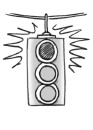Carefully examine each diagram below and explain why the geometric figure cannot exist. Support your statements with reasons. If a line looks straight, assume that it is. Homework Help ✎

1.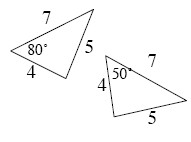2.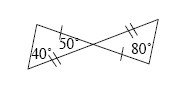3.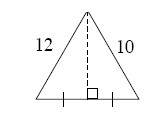4.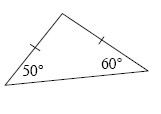5.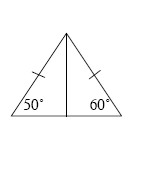6.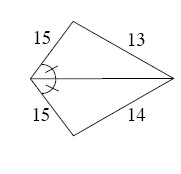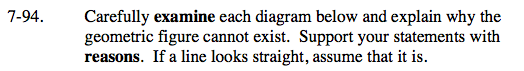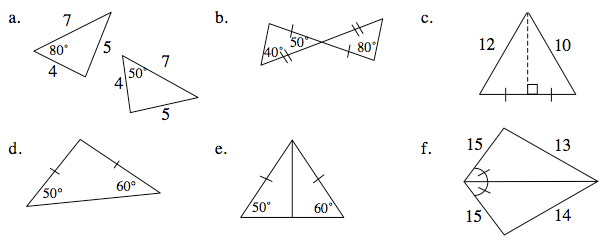Review the triangle congruence conjectures from the Math Notes box from Lesson 6.1.3.

What do you know about isosceles triangles?

The triangles should be congruent by SSS ≅, but 80° ≠ 50° and in congruent triangles all angles are equal as well.

The triangles should be congruent by SAS ≅, but 80° ≠ 90° and 40° ≠ 50° and in congruent triangles all angles are equal as well.

The triangles should be congruent by SAS ≅, but 10 ≠ 12 and in congruent triangles all sides are congruent as well.